Скачать презентацию regress ivreg generate egen by encode count collapse

a28d42d6bc49eafb8bbf8fed0d601792.ppt

• Количество слайдов: 49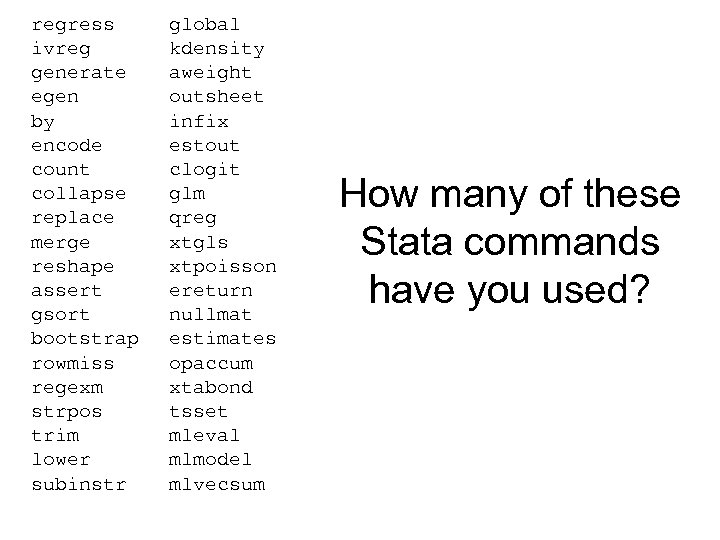regress ivreg generate egen by encode count collapse replace merge reshape assert gsort bootstrap rowmiss regexm strpos trim lower subinstr global kdensity aweight outsheet infix estout clogit glm qreg xtgls xtpoisson ereturn nullmat estimates opaccum xtabond tsset mleval mlmodel mlvecsum How many of these Stata commands have you used?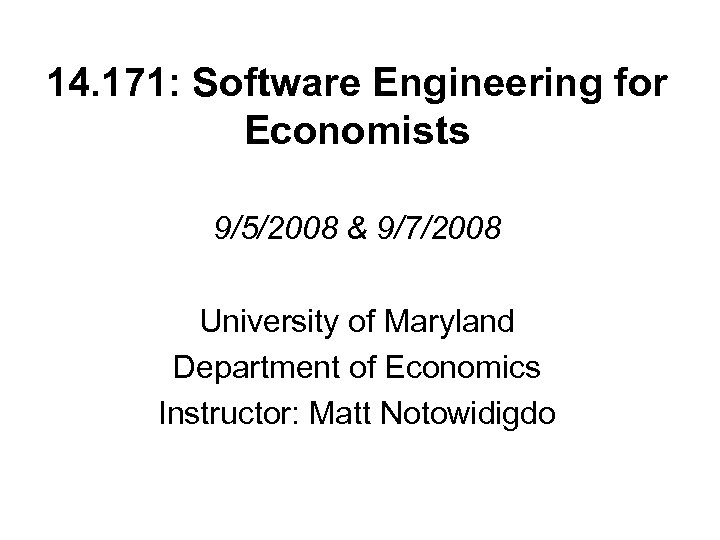14. 171: Software Engineering for Economists 9/5/2008 & 9/7/2008 University of Maryland Department of Economics Instructor: Matt Notowidigdo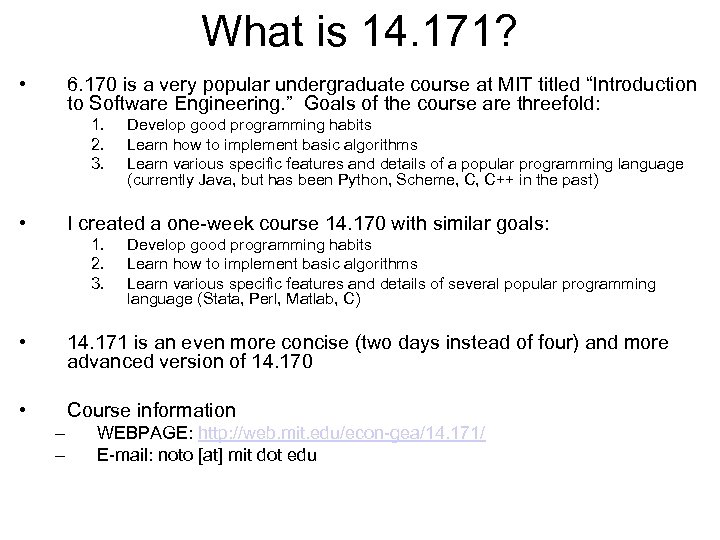What is 14. 171? • 6. 170 is a very popular undergraduate course at MIT titled “Introduction to Software Engineering. ” Goals of the course are threefold: 1. 2. 3. • Develop good programming habits Learn how to implement basic algorithms Learn various specific features and details of a popular programming language (currently Java, but has been Python, Scheme, C, C++ in the past) I created a one-week course 14. 170 with similar goals: 1. 2. 3. Develop good programming habits Learn how to implement basic algorithms Learn various specific features and details of several popular programming language (Stata, Perl, Matlab, C) • 14. 171 is an even more concise (two days instead of four) and more advanced version of 14. 170 • Course information – – WEBPAGE: http: //web. mit. edu/econ-gea/14. 171/ E-mail: noto [at] mit dot edu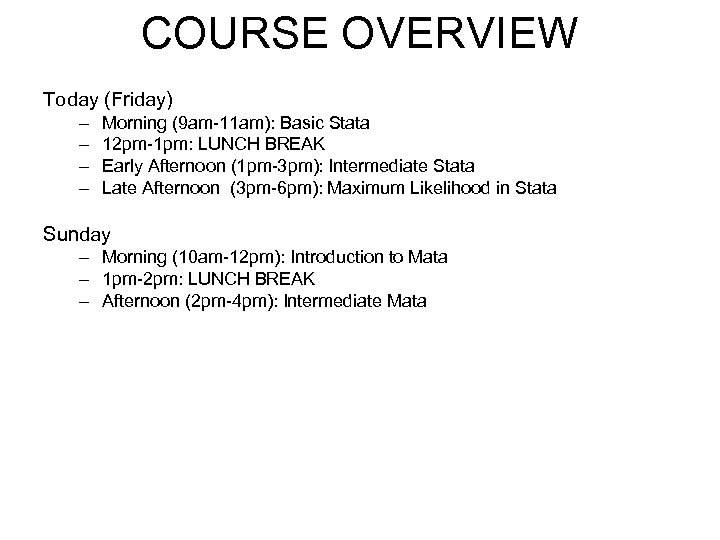COURSE OVERVIEW Today (Friday) – – Morning (9 am-11 am): Basic Stata 12 pm-1 pm: LUNCH BREAK Early Afternoon (1 pm-3 pm): Intermediate Stata Late Afternoon (3 pm-6 pm): Maximum Likelihood in Stata Sunday – Morning (10 am-12 pm): Introduction to Mata – 1 pm-2 pm: LUNCH BREAK – Afternoon (2 pm-4 pm): Intermediate Mata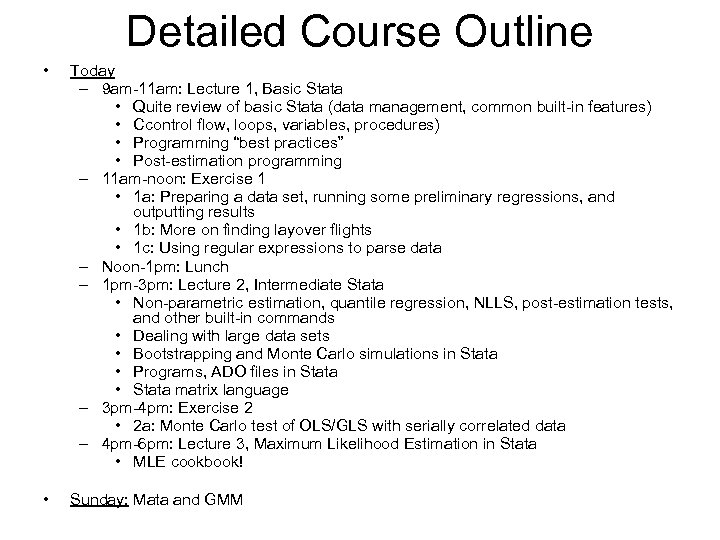Detailed Course Outline • Today – 9 am-11 am: Lecture 1, Basic Stata • Quite review of basic Stata (data management, common built-in features) • Ccontrol flow, loops, variables, procedures) • Programming “best practices” • Post-estimation programming – 11 am-noon: Exercise 1 • 1 a: Preparing a data set, running some preliminary regressions, and outputting results • 1 b: More on finding layover flights • 1 c: Using regular expressions to parse data – Noon-1 pm: Lunch – 1 pm-3 pm: Lecture 2, Intermediate Stata • Non-parametric estimation, quantile regression, NLLS, post-estimation tests, and other built-in commands • Dealing with large data sets • Bootstrapping and Monte Carlo simulations in Stata • Programs, ADO files in Stata • Stata matrix language – 3 pm-4 pm: Exercise 2 • 2 a: Monte Carlo test of OLS/GLS with serially correlated data – 4 pm-6 pm: Lecture 3, Maximum Likelihood Estimation in Stata • MLE cookbook! • Sunday: Mata and GMM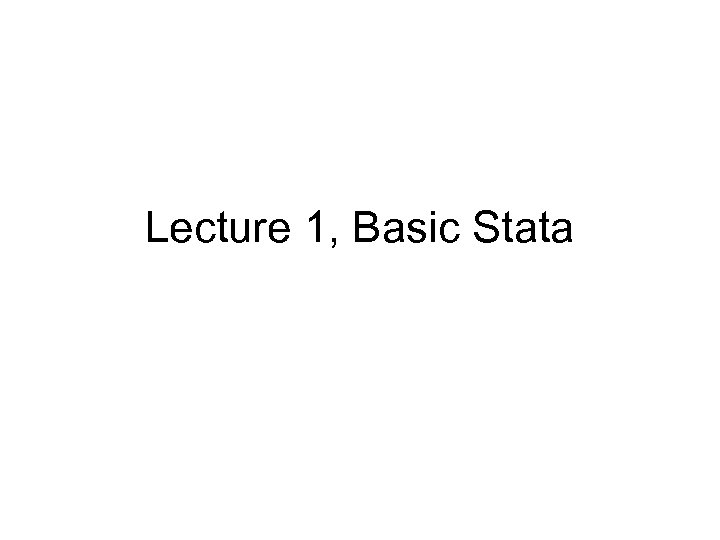Lecture 1, Basic Stata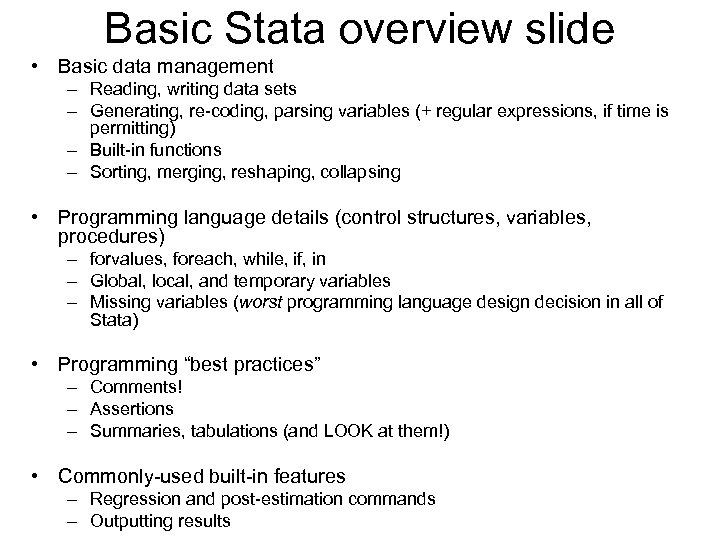Basic Stata overview slide • Basic data management – Reading, writing data sets – Generating, re-coding, parsing variables (+ regular expressions, if time is permitting) – Built-in functions – Sorting, merging, reshaping, collapsing • Programming language details (control structures, variables, procedures) – forvalues, foreach, while, if, in – Global, local, and temporary variables – Missing variables (worst programming language design decision in all of Stata) • Programming “best practices” – Comments! – Assertions – Summaries, tabulations (and LOOK at them!) • Commonly-used built-in features – Regression and post-estimation commands – Outputting results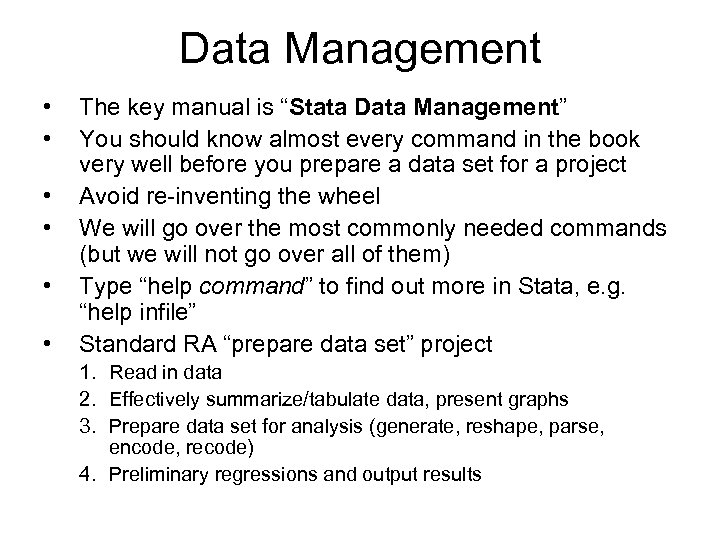Data Management • • • The key manual is “Stata Data Management” You should know almost every command in the book very well before you prepare a data set for a project Avoid re-inventing the wheel We will go over the most commonly needed commands (but we will not go over all of them) Type “help command” to find out more in Stata, e. g. “help infile” Standard RA “prepare data set” project 1. Read in data 2. Effectively summarize/tabulate data, present graphs 3. Prepare data set for analysis (generate, reshape, parse, encode, recode) 4. Preliminary regressions and output results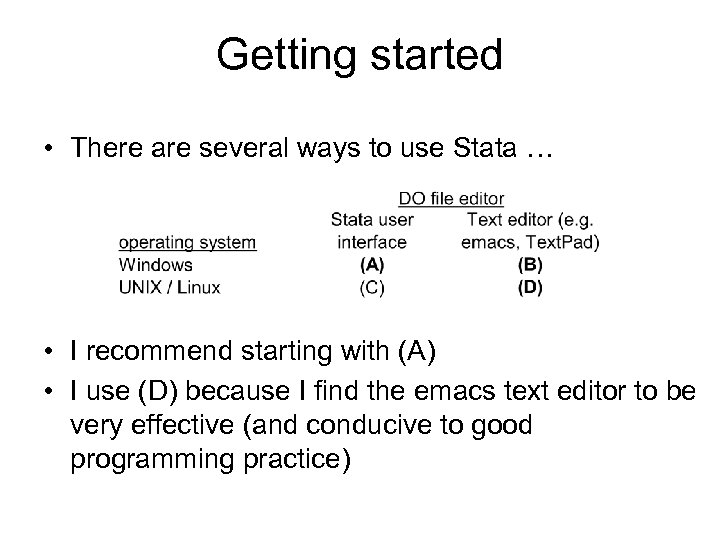Getting started • There are several ways to use Stata … • I recommend starting with (A) • I use (D) because I find the emacs text editor to be very effective (and conducive to good programming practice)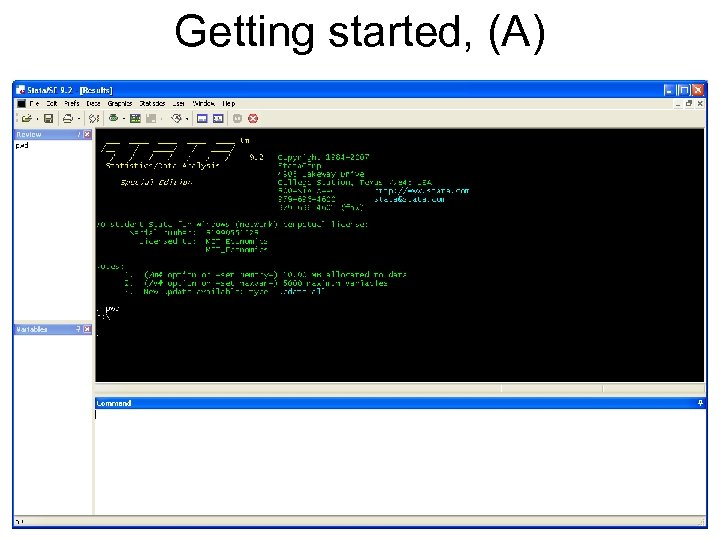Getting started, (A)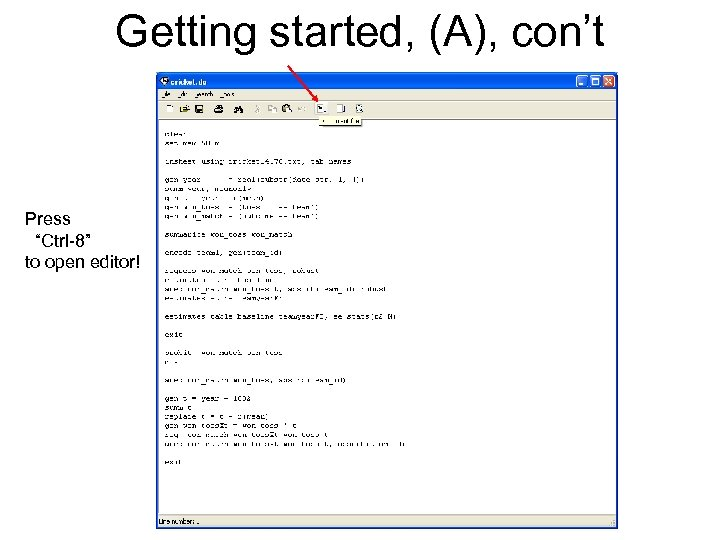Getting started, (A), con’t Press “Ctrl-8” to open editor!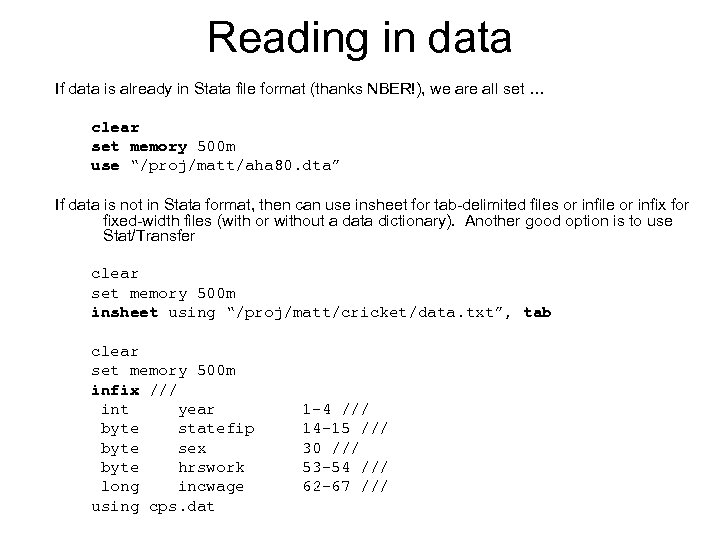Reading in data If data is already in Stata file format (thanks NBER!), we are all set … clear set memory 500 m use “/proj/matt/aha 80. dta” If data is not in Stata format, then can use insheet for tab-delimited files or infile or infix for fixed-width files (with or without a data dictionary). Another good option is to use Stat/Transfer clear set memory 500 m insheet using “/proj/matt/cricket/data. txt”, tab clear set memory 500 m infix /// int year byte statefip byte sex byte hrswork long incwage using cps. dat 1 -4 /// 14 -15 /// 30 /// 53 -54 /// 62 -67 ///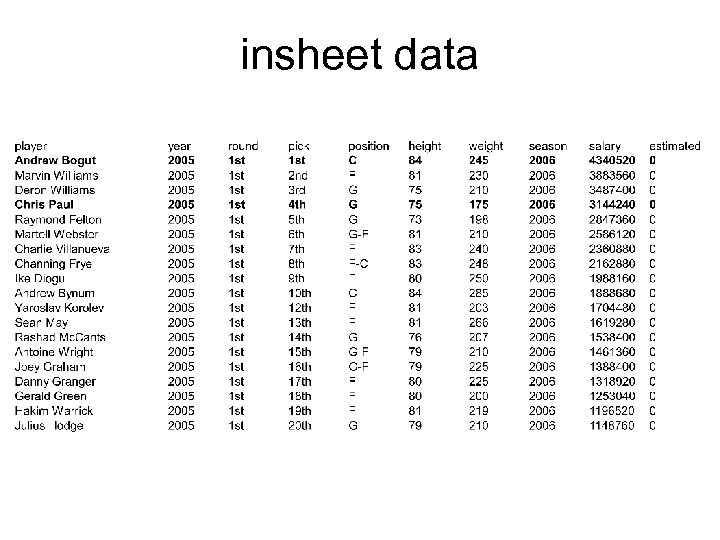insheet data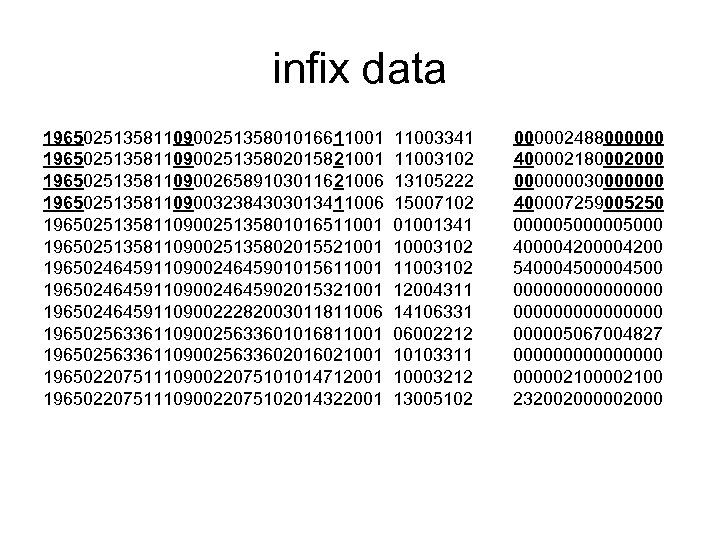infix data 1965025135811090025135801016611001 1965025135811090025135802015821001 1965025135811090026589103011621006 1965025135811090032384303013411006 1965025135811090025135801016511001 1965025135811090025135802015521001 1965024645911090024645901015611001 1965024645911090024645902015321001 1965024645911090022282003011811006 1965025633611090025633601016811001 1965025633611090025633602016021001 1965022075111090022075101014712001 1965022075111090022075102014322001 11003341 11003102 13105222 15007102 01001341 10003102 11003102 12004311 14106331 06002212 10103311 10003212 13005102 000002488000000 400002180002000 000000030000000 400007259005250 000005000 400004200 540004500 0000000000000005067004827 000000002100 232002000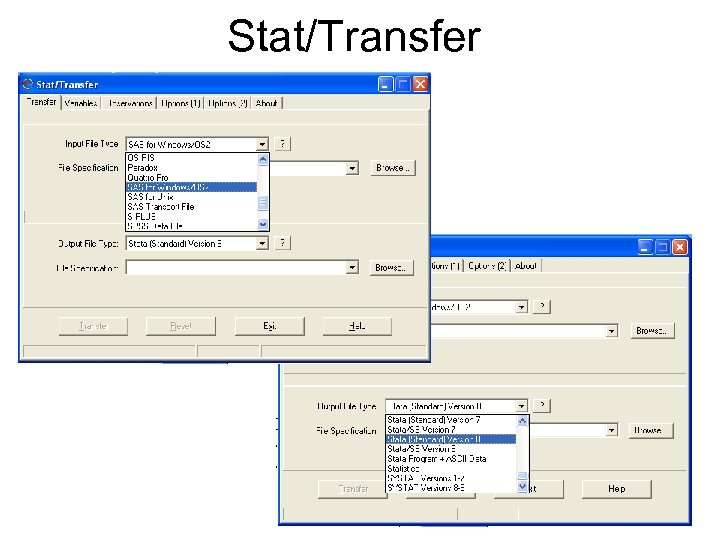Stat/Transfer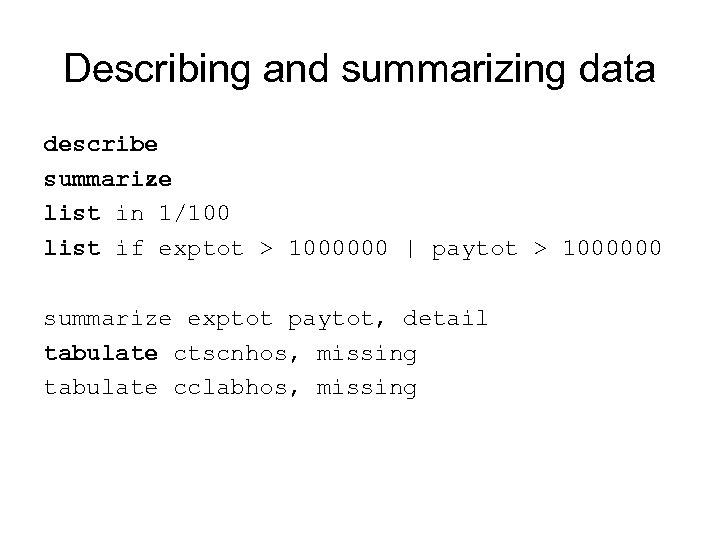Describing and summarizing data describe summarize list in 1/100 list if exptot > 1000000 | paytot > 1000000 summarize exptot paytot, detail tabulate ctscnhos, missing tabulate cclabhos, missing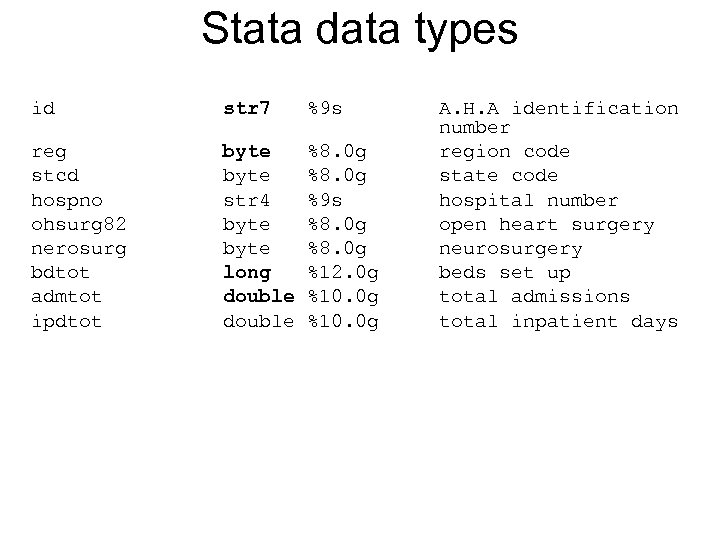Stata data types id str 7 %9 s reg stcd hospno ohsurg 82 nerosurg bdtot admtot ipdtot byte str 4 byte long double %8. 0 g %9 s %8. 0 g %12. 0 g %10. 0 g A. H. A identification number region code state code hospital number open heart surgery neurosurgery beds set up total admissions total inpatient days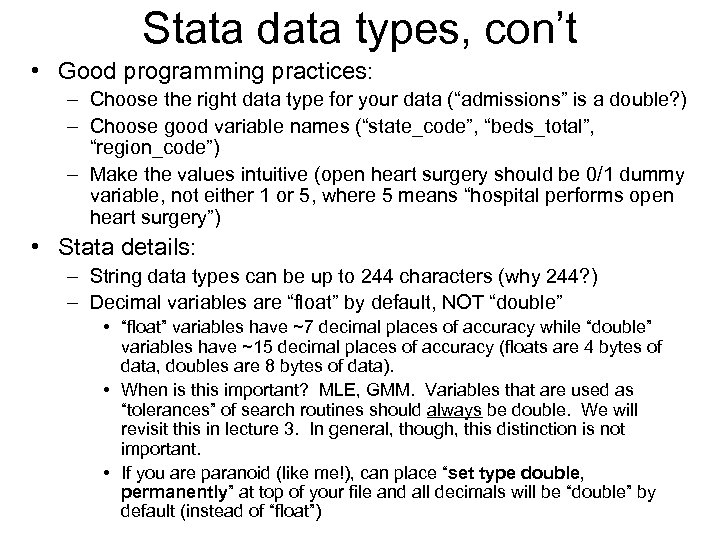Stata data types, con’t • Good programming practices: – Choose the right data type for your data (“admissions” is a double? ) – Choose good variable names (“state_code”, “beds_total”, “region_code”) – Make the values intuitive (open heart surgery should be 0/1 dummy variable, not either 1 or 5, where 5 means “hospital performs open heart surgery”) • Stata details: – String data types can be up to 244 characters (why 244? ) – Decimal variables are “float” by default, NOT “double” • “float” variables have ~7 decimal places of accuracy while “double” variables have ~15 decimal places of accuracy (floats are 4 bytes of data, doubles are 8 bytes of data). • When is this important? MLE, GMM. Variables that are used as “tolerances” of search routines should always be double. We will revisit this in lecture 3. In general, though, this distinction is not important. • If you are paranoid (like me!), can place “set type double, permanently” at top of your file and all decimals will be “double” by default (instead of “float”)Summarizing data • Why only 6420 observations for “fyr” variable? 0 observations for “id” variable? • Are there any missing “id” variables? How could we tell? • How many observations are in the data set?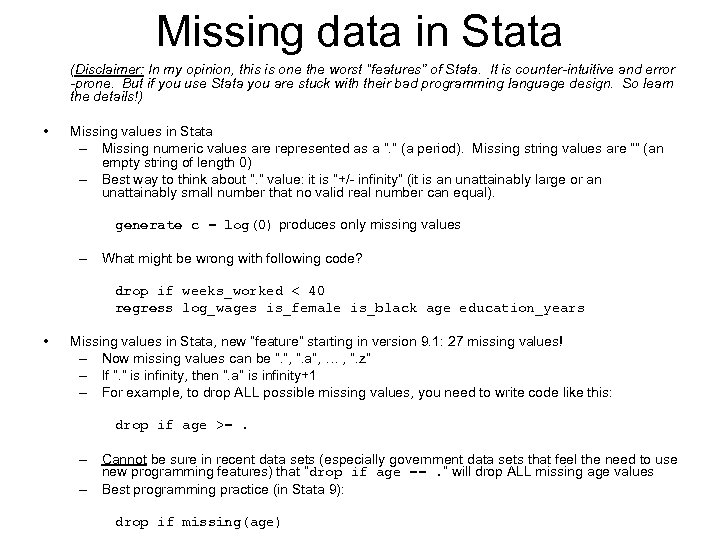Missing data in Stata (Disclaimer: In my opinion, this is one the worst “features” of Stata. It is counter-intuitive and error -prone. But if you use Stata you are stuck with their bad programming language design. So learn the details!) • Missing values in Stata – Missing numeric values are represented as a “. ” (a period). Missing string values are “” (an empty string of length 0) – Best way to think about “. ” value: it is “+/- infinity” (it is an unattainably large or an unattainably small number that no valid real number can equal). generate c = log(0) produces only missing values – What might be wrong with following code? drop if weeks_worked < 40 regress log_wages is_female is_black age education_years • Missing values in Stata, new “feature” starting in version 9. 1: 27 missing values! – Now missing values can be “. ”, “. a”, … , “. z” – If “. ” is infinity, then “. a” is infinity+1 – For example, to drop ALL possible missing values, you need to write code like this: drop if age >=. – Cannot be sure in recent data sets (especially government data sets that feel the need to use new programming features) that “drop if age ==. ” will drop ALL missing age values – Best programming practice (in Stata 9): drop if missing(age)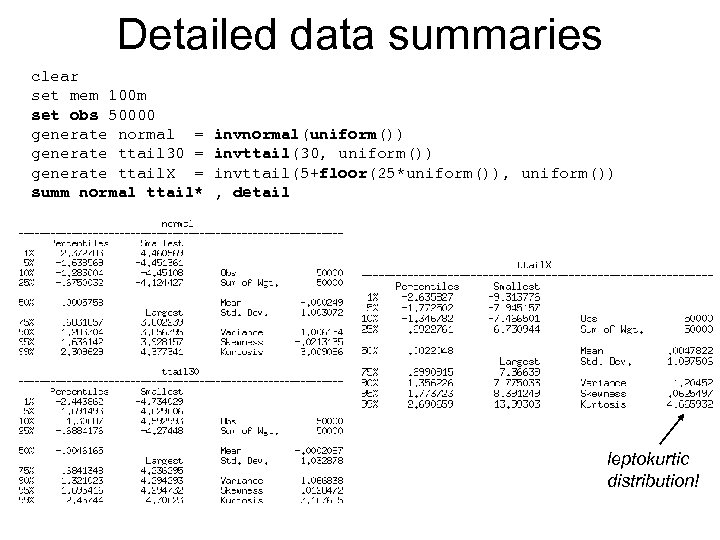Detailed data summaries clear set mem 100 m set obs 50000 generate normal = generate ttail 30 = generate ttail. X = summ normal ttail* invnormal(uniform()) invttail(30, uniform()) invttail(5+floor(25*uniform()), uniform()) , detail leptokurtic distribution!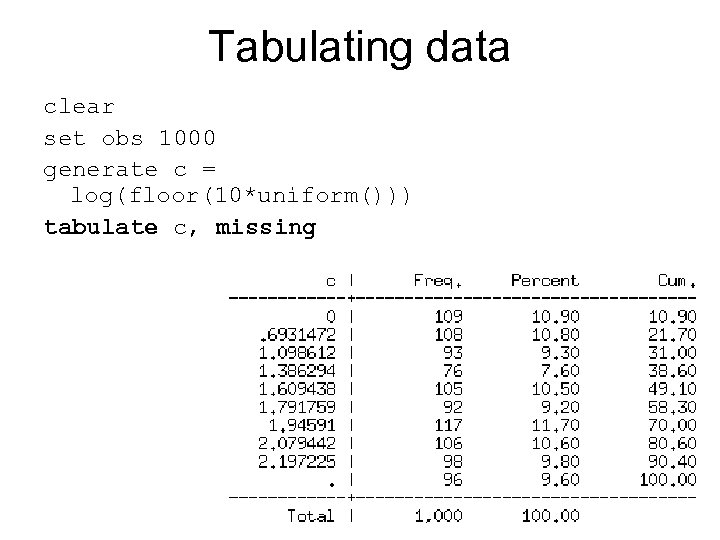Tabulating data clear set obs 1000 generate c = log(floor(10*uniform())) tabulate c, missing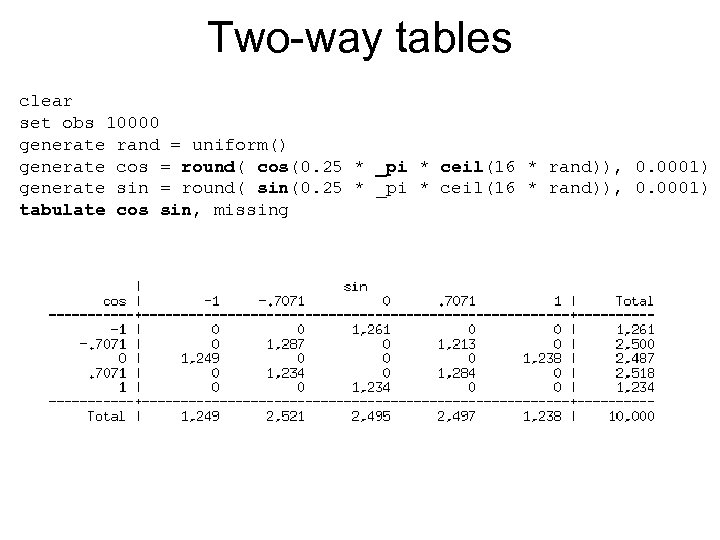Two-way tables clear set obs 10000 generate rand = uniform() generate cos = round( cos(0. 25 * _pi * ceil(16 * rand)), 0. 0001) generate sin = round( sin(0. 25 * _pi * ceil(16 * rand)), 0. 0001) tabulate cos sin, missing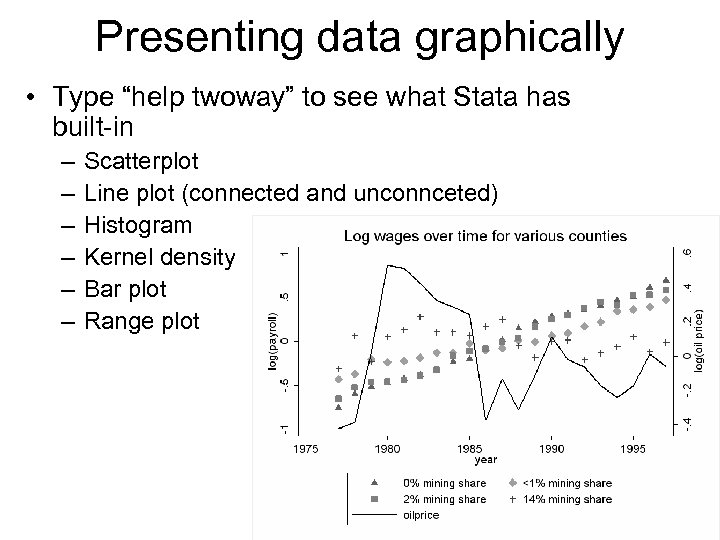Presenting data graphically • Type “help twoway” to see what Stata has built-in – – – Scatterplot Line plot (connected and unconnceted) Histogram Kernel density Bar plot Range plot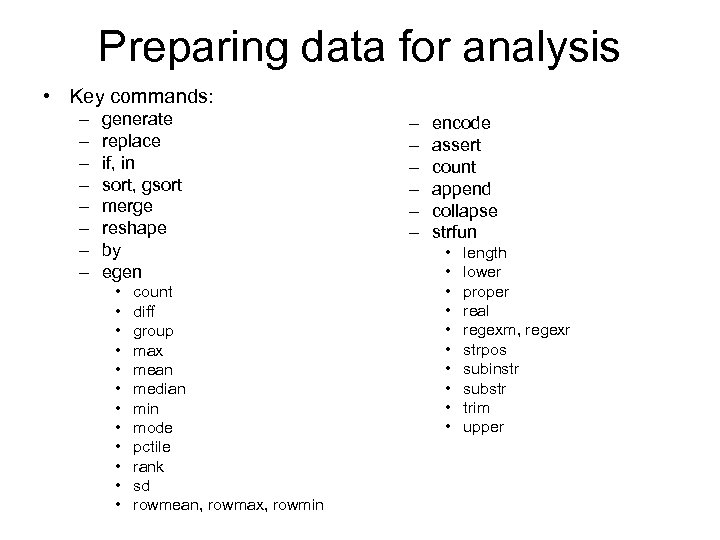Preparing data for analysis • Key commands: – – – – generate replace if, in sort, gsort merge reshape by egen • • • count diff group max mean median min mode pctile rank sd rowmean, rowmax, rowmin – – – encode assert count append collapse strfun • • • length lower proper real regexm, regexr strpos subinstr substr trim upper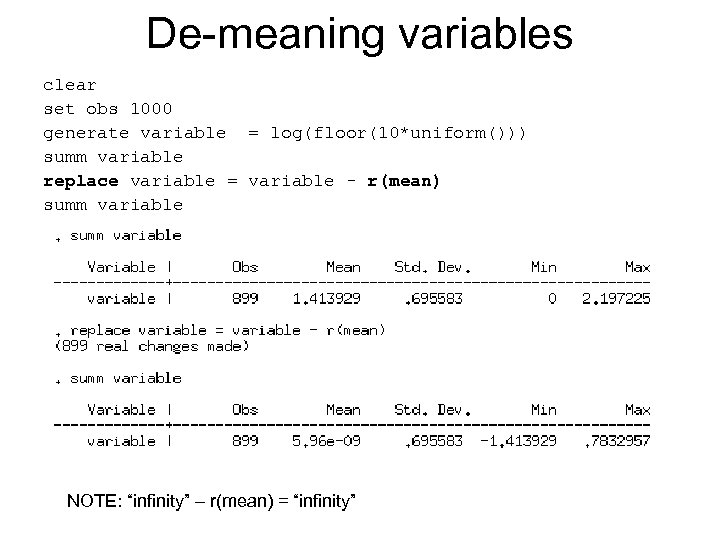De-meaning variables clear set obs 1000 generate variable = log(floor(10*uniform())) summ variable replace variable = variable - r(mean) summ variable NOTE: “infinity” – r(mean) = “infinity”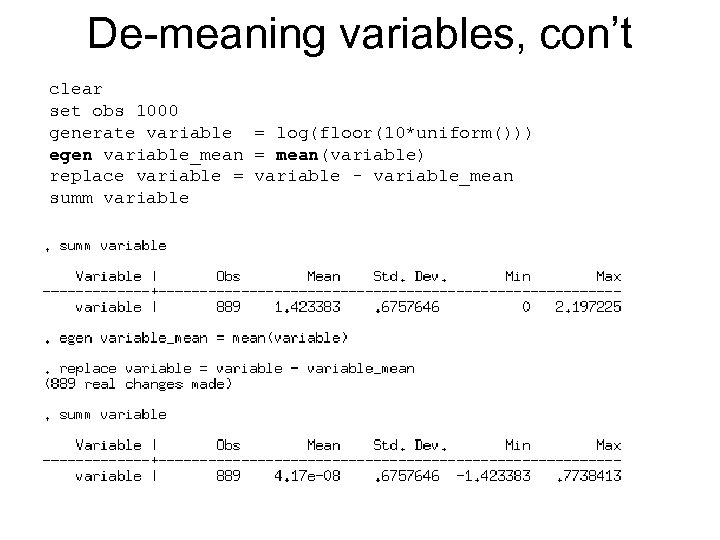De-meaning variables, con’t clear set obs 1000 generate variable = log(floor(10*uniform())) egen variable_mean = mean(variable) replace variable = variable - variable_mean summ variable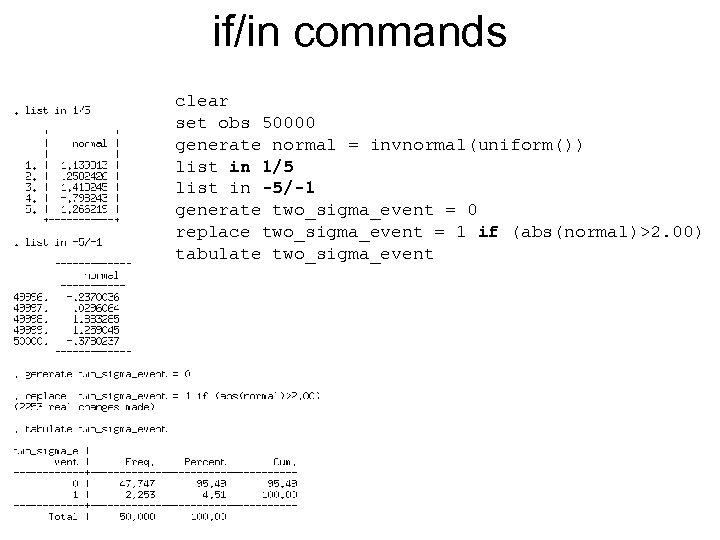if/in commands clear set obs 50000 generate normal = invnormal(uniform()) list in 1/5 list in -5/-1 generate two_sigma_event = 0 replace two_sigma_event = 1 if (abs(normal)>2. 00) tabulate two_sigma_event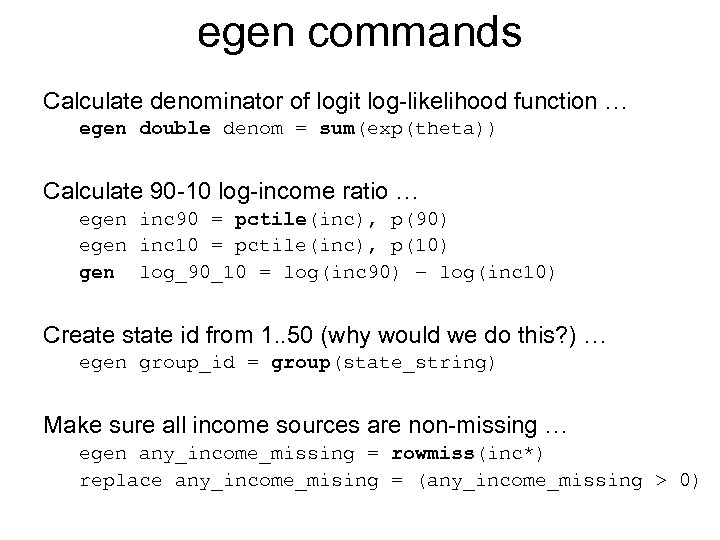egen commands Calculate denominator of logit log-likelihood function … egen double denom = sum(exp(theta)) Calculate 90 -10 log-income ratio … egen inc 90 = pctile(inc), p(90) egen inc 10 = pctile(inc), p(10) gen log_90_10 = log(inc 90) – log(inc 10) Create state id from 1. . 50 (why would we do this? ) … egen group_id = group(state_string) Make sure all income sources are non-missing … egen any_income_missing = rowmiss(inc*) replace any_income_mising = (any_income_missing > 0)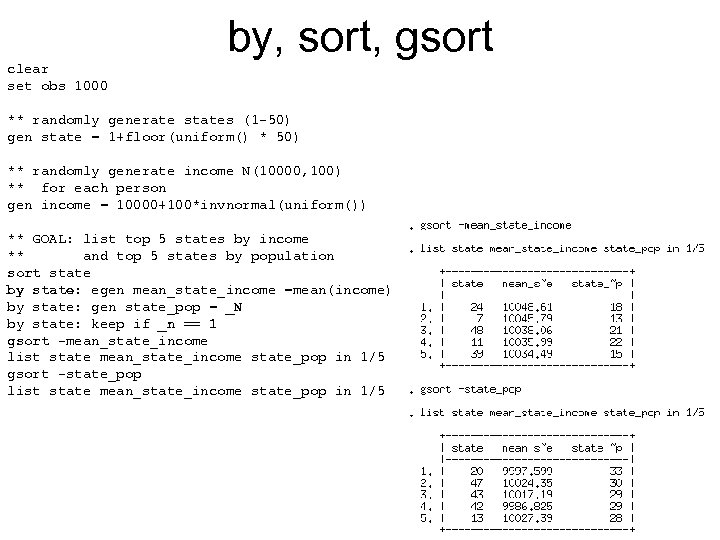by, sort, gsort clear set obs 1000 ** randomly generate states (1 -50) gen state = 1+floor(uniform() * 50) ** randomly generate income N(10000, 100) ** for each person gen income = 10000+100*invnormal(uniform()) ** GOAL: list top 5 states by income ** and top 5 states by population sort state by state: egen mean_state_income =mean(income) by state: gen state_pop = _N by state: keep if _n == 1 gsort -mean_state_income list state mean_state_income state_pop in 1/5 gsort -state_pop list state mean_state_income state_pop in 1/5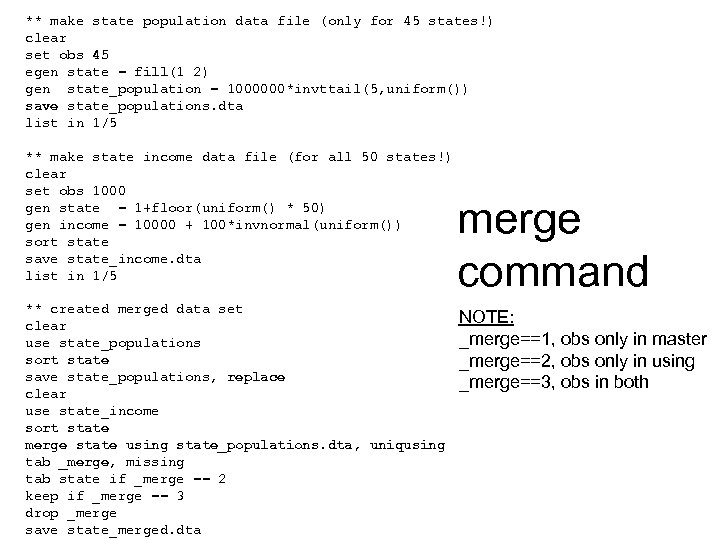** make state population data file (only for 45 states!) clear set obs 45 egen state = fill(1 2) gen state_population = 1000000*invttail(5, uniform()) save state_populations. dta list in 1/5 ** make state income data file (for all 50 states!) clear set obs 1000 gen state = 1+floor(uniform() * 50) gen income = 10000 + 100*invnormal(uniform()) sort state save state_income. dta list in 1/5 ** created merged data set clear use state_populations sort state save state_populations, replace clear use state_income sort state merge state using state_populations. dta, uniqusing tab _merge, missing tab state if _merge == 2 keep if _merge == 3 drop _merge save state_merged. dta merge command NOTE: _merge==1, obs only in master _merge==2, obs only in using _merge==3, obs in both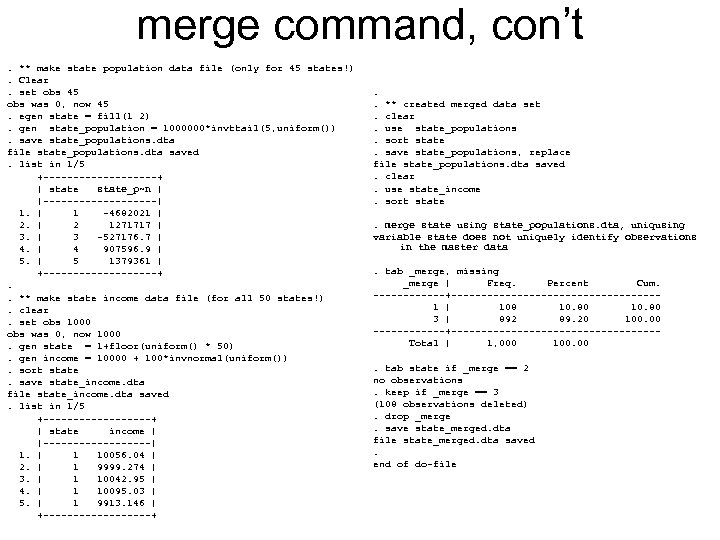merge command, con’t. ** make state population data file (only for 45 states!). Clear. set obs 45 obs was 0, now 45. egen state = fill(1 2). gen state_population = 1000000*invttail(5, uniform()). save state_populations. dta file state_populations. dta saved. list in 1/5 +----------+ | state_p~n | |----------| 1. | 1 -4682021 | 2 1271717 | 3 -527176. 7 | 4 907596. 9 | 5 1379361 | +----------+. . ** make state income data file (for all 50 states!). clear. set obs 1000 obs was 0, now 1000. gen state = 1+floor(uniform() * 50). gen income = 10000 + 100*invnormal(uniform()). sort state. save state_income. dta file state_income. dta saved. list in 1/5 +---------+ | state income | |---------| 1. | 1 10056. 04 | 2. | 1 9999. 274 | 3. | 1 10042. 95 | 4. | 1 10095. 03 | 5. | 1 9913. 146 | +---------+ . . ** created merged data set. clear. use state_populations. sort state. save state_populations, replace file state_populations. dta saved. clear. use state_income. sort state. merge state using state_populations. dta, uniqusing variable state does not uniquely identify observations in the master data. tab _merge, missing _merge | Freq. Percent Cum. ------+-----------------1 | 108 10. 80 3 | 892 89. 20 100. 00 ------+-----------------Total | 1, 000 100. tab state if _merge == 2 no observations. keep if _merge == 3 (108 observations deleted). drop _merge. save state_merged. dta file state_merged. dta saved. end of do-file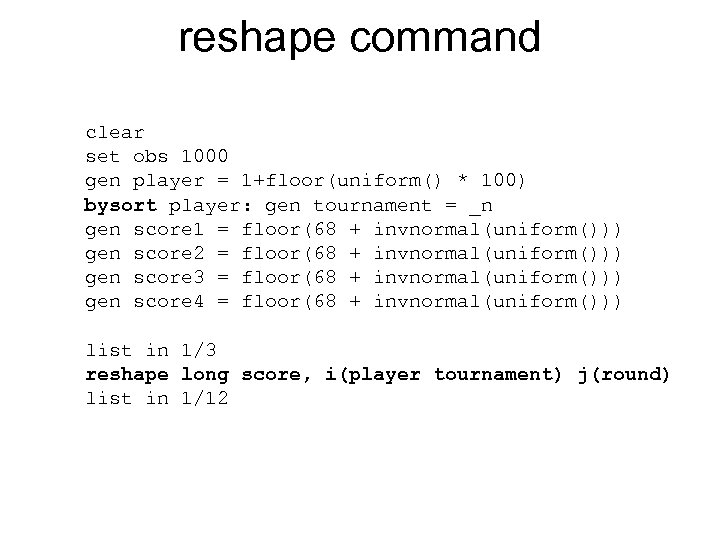reshape command clear set obs 1000 gen player = 1+floor(uniform() * 100) bysort player: gen tournament = _n gen score 1 = floor(68 + invnormal(uniform())) gen score 2 = floor(68 + invnormal(uniform())) gen score 3 = floor(68 + invnormal(uniform())) gen score 4 = floor(68 + invnormal(uniform())) list in 1/3 reshape long score, i(player tournament) j(round) list in 1/12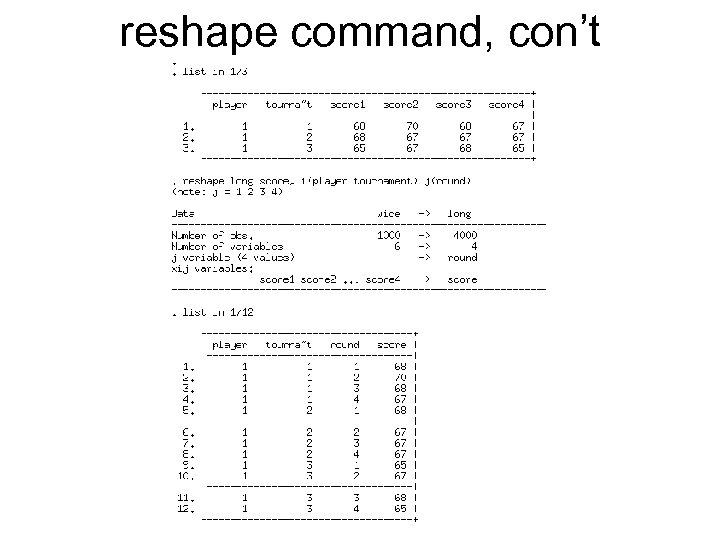reshape command, con’t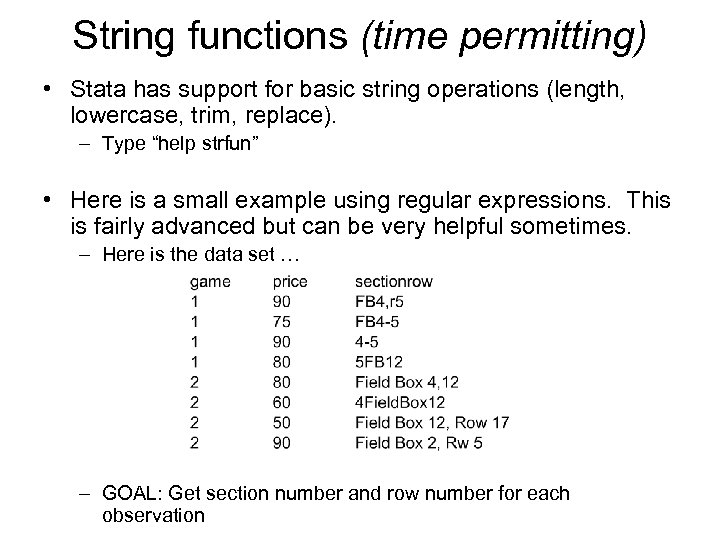String functions (time permitting) • Stata has support for basic string operations (length, lowercase, trim, replace). – Type “help strfun” • Here is a small example using regular expressions. This is fairly advanced but can be very helpful sometimes. – Here is the data set … – GOAL: Get section number and row number for each observation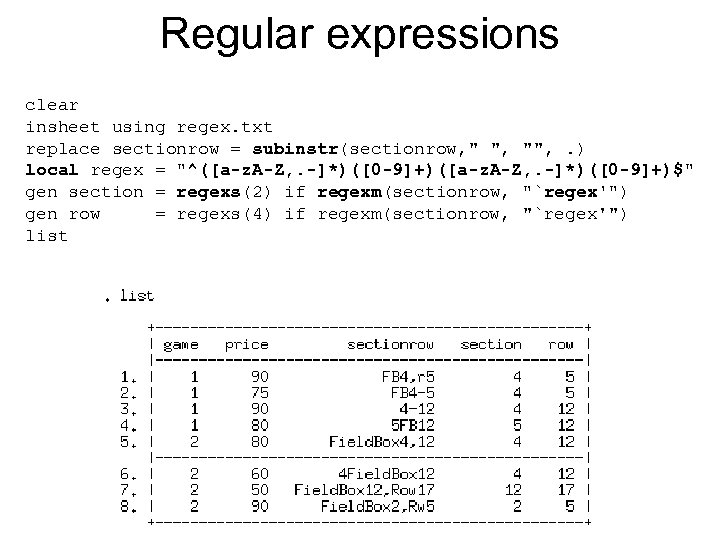Regular expressions clear insheet using regex. txt replace sectionrow = subinstr(sectionrow, " ", "", . ) local regex = "^([a-z. A-Z, . -]*)([0 -9]+)\$" gen section = regexs(2) if regexm(sectionrow, "`regex'") gen row = regexs(4) if regexm(sectionrow, "`regex'") list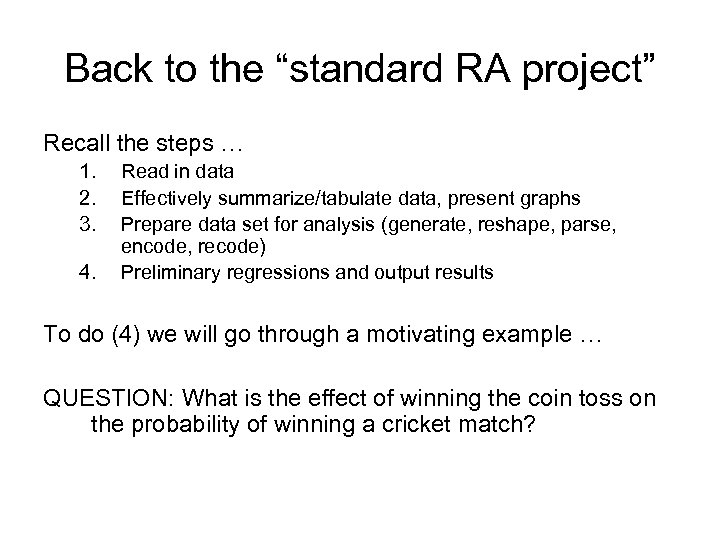Back to the “standard RA project” Recall the steps … 1. 2. 3. 4. Read in data Effectively summarize/tabulate data, present graphs Prepare data set for analysis (generate, reshape, parse, encode, recode) Preliminary regressions and output results To do (4) we will go through a motivating example … QUESTION: What is the effect of winning the coin toss on the probability of winning a cricket match?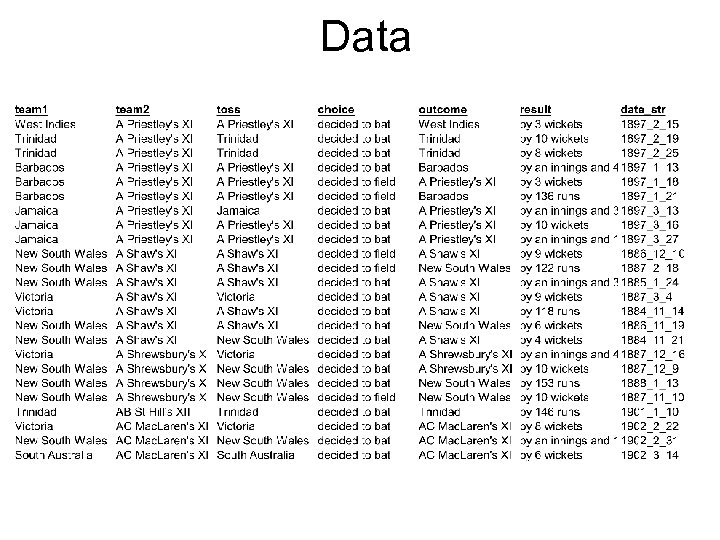Data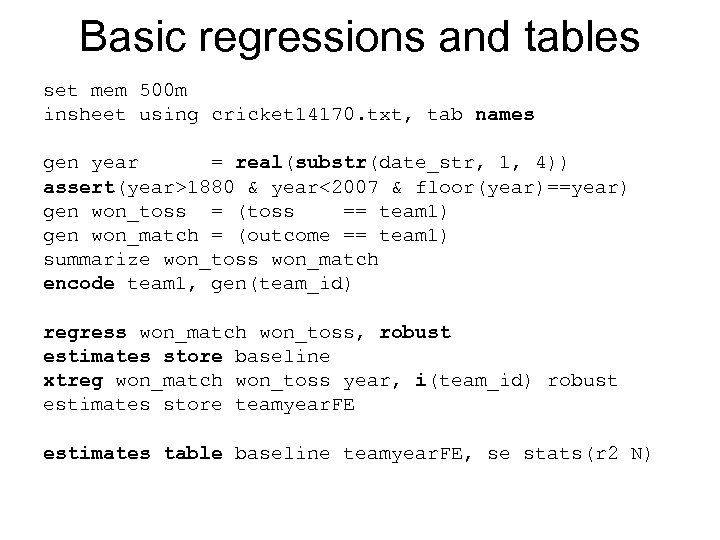Basic regressions and tables set mem 500 m insheet using cricket 14170. txt, tab names gen year = real(substr(date_str, 1, 4)) assert(year>1880 & year<2007 & floor(year)==year) gen won_toss = (toss == team 1) gen won_match = (outcome == team 1) summarize won_toss won_match encode team 1, gen(team_id) regress won_match won_toss, robust estimates store baseline xtreg won_match won_toss year, i(team_id) robust estimates store teamyear. FE estimates table baseline teamyear. FE, se stats(r 2 N)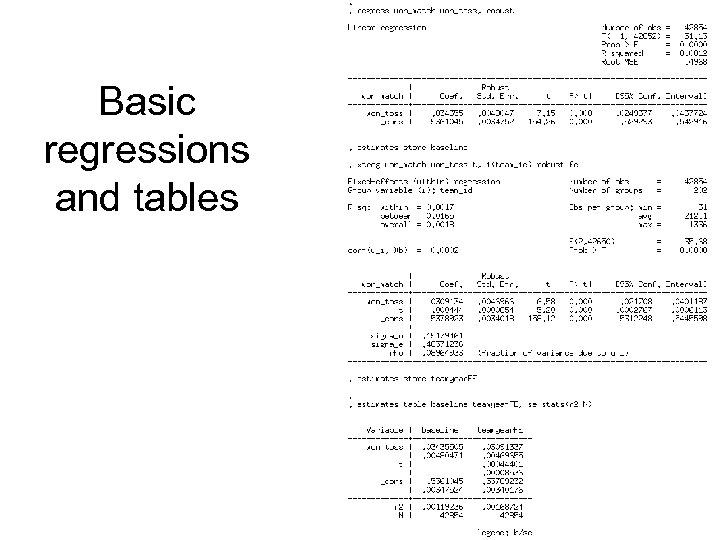Basic regressions and tables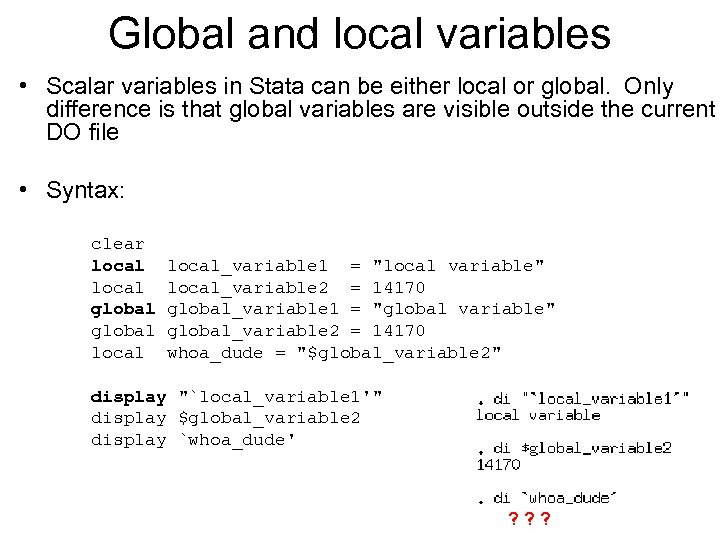Global and local variables • Scalar variables in Stata can be either local or global. Only difference is that global variables are visible outside the current DO file • Syntax: clear local global local_variable 1 = "local variable" local_variable 2 = 14170 global_variable 1 = "global variable" global_variable 2 = 14170 whoa_dude = "\$global_variable 2" display "`local_variable 1'" display \$global_variable 2 display `whoa_dude' ? ? ?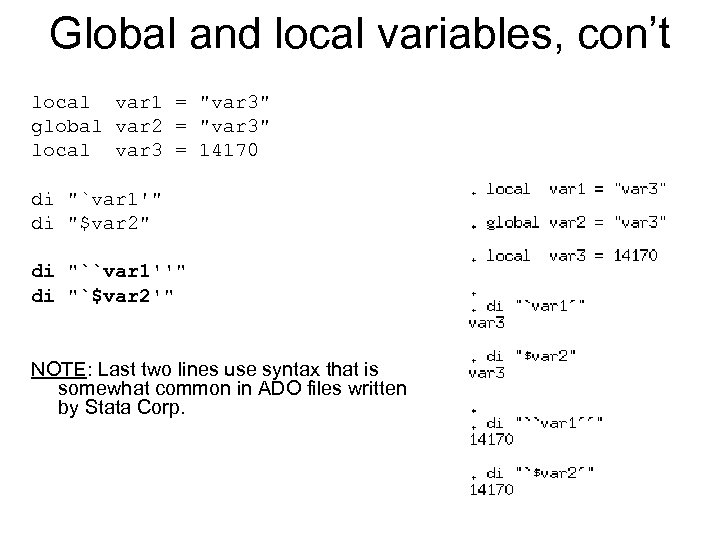Global and local variables, con’t local var 1 = "var 3" global var 2 = "var 3" local var 3 = 14170 di "`var 1'" di "\$var 2" di "``var 1''" di "`\$var 2'" NOTE: Last two lines use syntax that is somewhat common in ADO files written by Stata Corp.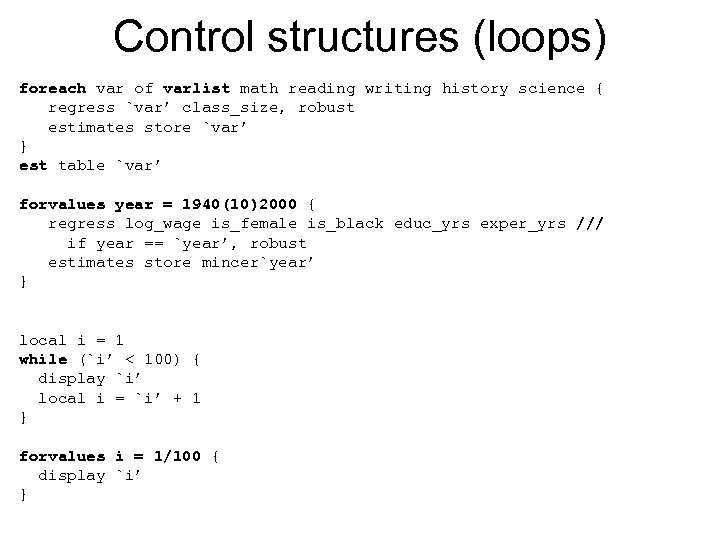Control structures (loops) foreach var of varlist math reading writing history science { regress `var’ class_size, robust estimates store `var’ } est table `var’ forvalues year = 1940(10)2000 { regress log_wage is_female is_black educ_yrs exper_yrs /// if year == `year’, robust estimates store mincer`year’ } local i = 1 while (`i’ < 100) { display `i’ local i = `i’ + 1 } forvalues i = 1/100 { display `i’ }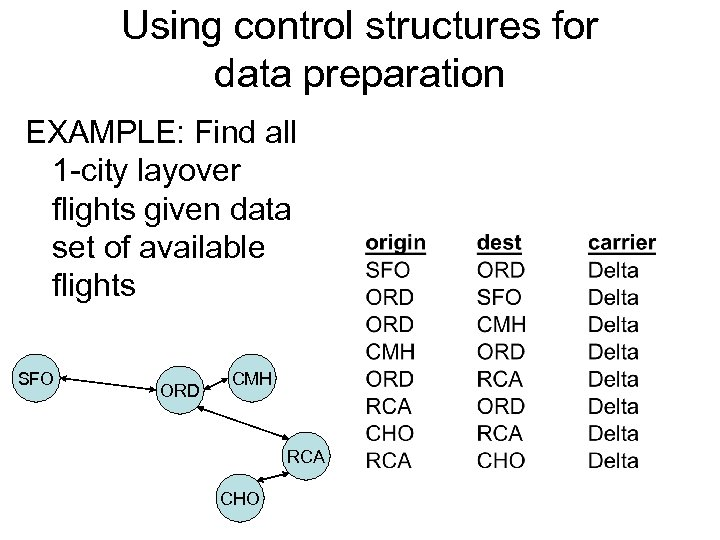Using control structures for data preparation EXAMPLE: Find all 1 -city layover flights given data set of available flights SFO ORD CMH RCA CHO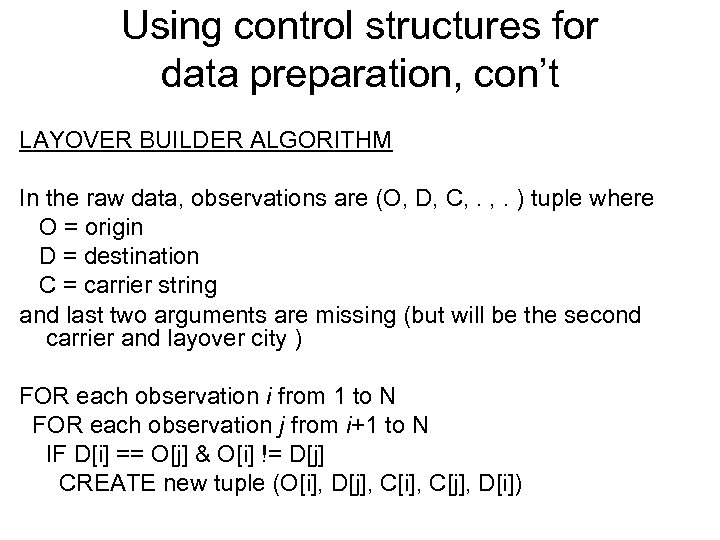Using control structures for data preparation, con’t LAYOVER BUILDER ALGORITHM In the raw data, observations are (O, D, C, . ) tuple where O = origin D = destination C = carrier string and last two arguments are missing (but will be the second carrier and layover city ) FOR each observation i from 1 to N FOR each observation j from i+1 to N IF D[i] == O[j] & O[i] != D[j] CREATE new tuple (O[i], D[j], C[i], C[j], D[i])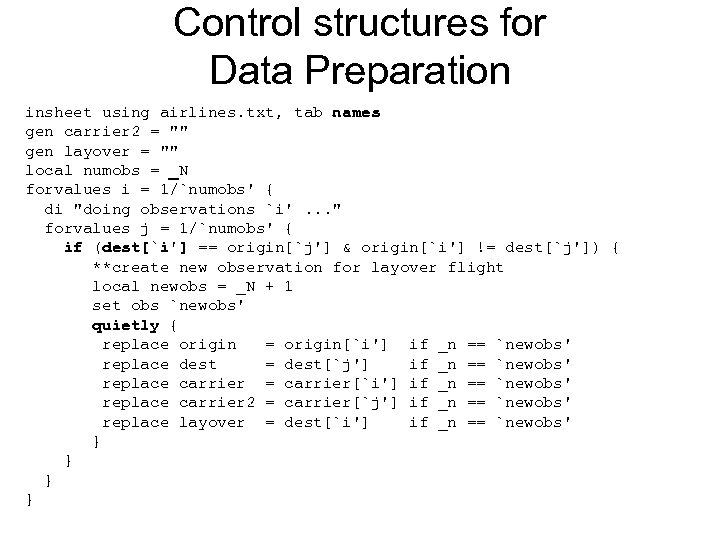Control structures for Data Preparation insheet using airlines. txt, tab names gen carrier 2 = "" gen layover = "" local numobs = _N forvalues i = 1/`numobs' { di "doing observations `i'. . . " forvalues j = 1/`numobs' { if (dest[`i'] == origin[`j'] & origin[`i'] != dest[`j']) { **create new observation for layover flight local newobs = _N + 1 set obs `newobs' quietly { replace origin = origin[`i'] if _n == `newobs' replace dest = dest[`j'] if _n == `newobs' replace carrier = carrier[`i'] if _n == `newobs' replace carrier 2 = carrier[`j'] if _n == `newobs' replace layover = dest[`i'] if _n == `newobs' } }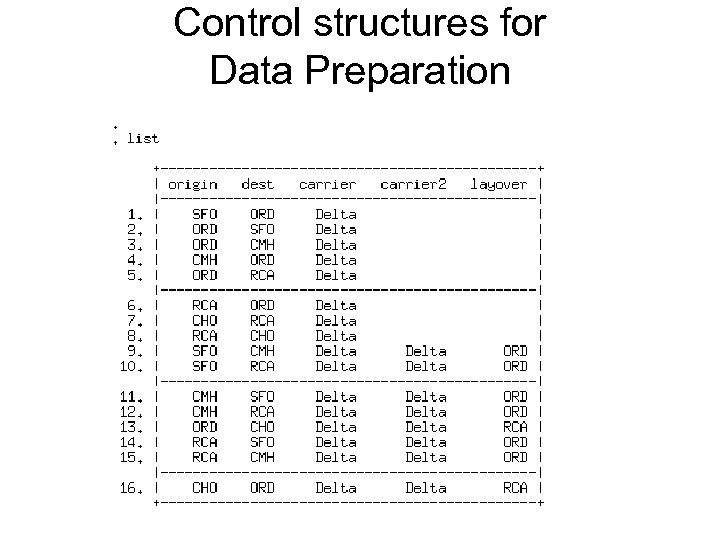Control structures for Data Preparation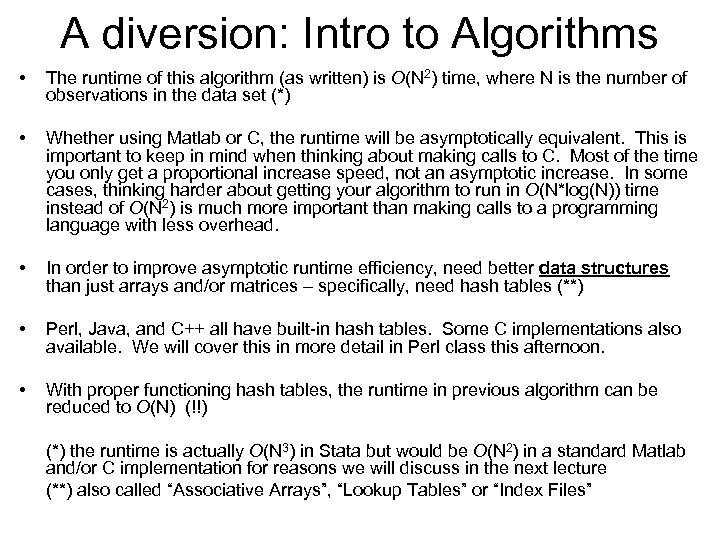A diversion: Intro to Algorithms • The runtime of this algorithm (as written) is O(N 2) time, where N is the number of observations in the data set (*) • Whether using Matlab or C, the runtime will be asymptotically equivalent. This is important to keep in mind when thinking about making calls to C. Most of the time you only get a proportional increase speed, not an asymptotic increase. In some cases, thinking harder about getting your algorithm to run in O(N*log(N)) time instead of O(N 2) is much more important than making calls to a programming language with less overhead. • In order to improve asymptotic runtime efficiency, need better data structures than just arrays and/or matrices – specifically, need hash tables (**) • Perl, Java, and C++ all have built-in hash tables. Some C implementations also available. We will cover this in more detail in Perl class this afternoon. • With proper functioning hash tables, the runtime in previous algorithm can be reduced to O(N) (!!) (*) the runtime is actually O(N 3) in Stata but would be O(N 2) in a standard Matlab and/or C implementation for reasons we will discuss in the next lecture (**) also called “Associative Arrays”, “Lookup Tables” or “Index Files”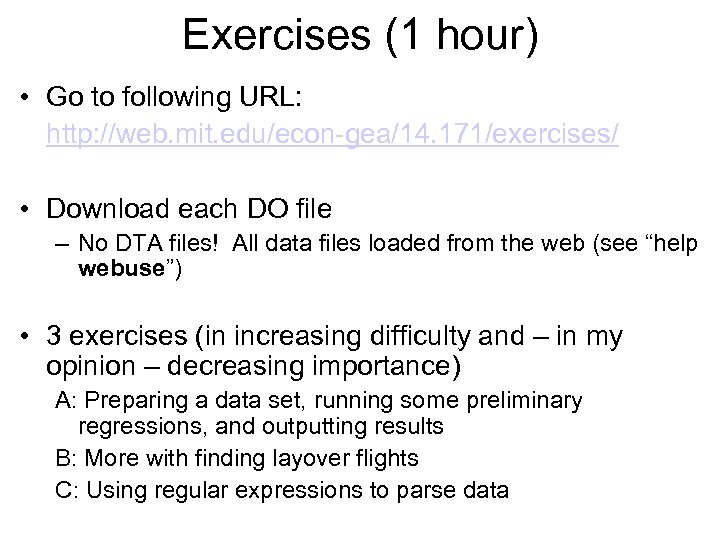Exercises (1 hour) • Go to following URL: http: //web. mit. edu/econ-gea/14. 171/exercises/ • Download each DO file – No DTA files! All data files loaded from the web (see “help webuse”) • 3 exercises (in increasing difficulty and – in my opinion – decreasing importance) A: Preparing a data set, running some preliminary regressions, and outputting results B: More with finding layover flights C: Using regular expressions to parse data